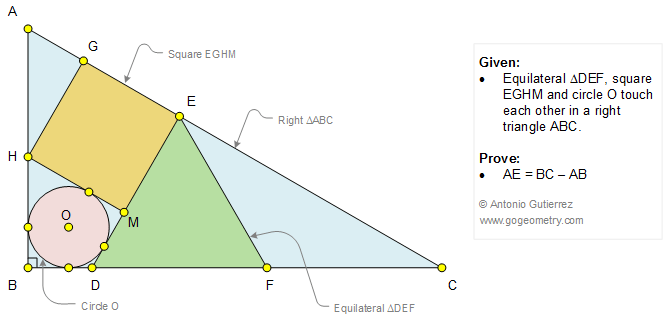#Problem 1092. Equilateral Triangle, Square, Circle, Tangent, 90 Degree, Sangaku

 < PREVIOUS PROBLEM  |  NEXT PROBLEM > An equilateral triangle DEF, a square EGHM and a circle O touch each other in a right triangle ABC (see the figure below). Prove that AE = BC - ABReference: Fukagawa Hidetoshi, Tony Rothman, Sacred Mathematics: Japanese Temple Geometry(Princenton University Press, 2008).

 Home | Geometry | Search | Problems | All Problems | Open Problems | Visual Index | 1091-1100 | Square | Equilateral Triangle | Triangles | Circle | Tangent Line | Sangaku | Post a solution / comment | by Antonio Gutierrez